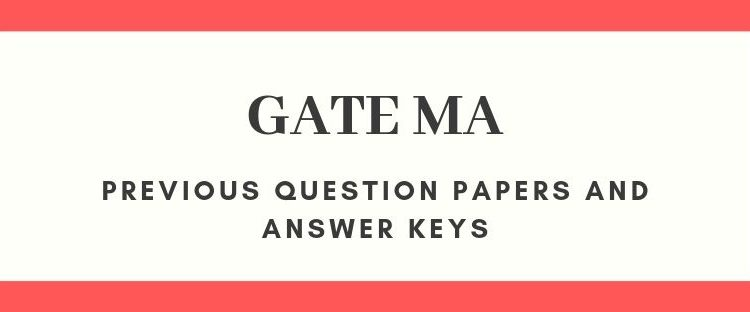GATE

# 12 Previous Year solved papers of GATE MA- Mathematics

### Last year question paper collection of GATE MathsGraduate Aptitude Test in Engineering (GATE) is a national level entrance examination for admissions to Post Graduation programs like M.Tech, M.Arch, ME, etc.

GATE Qualification is mandatory for seeking admission to:

(i) Master’s programs in Engineering/ Architecture/ Technology
(ii) Doctoral programs in relevant branches of Science.

The scores are accepted by more than 900+ institutes across India and is valid for three years post announcement of results.

GATE 2020 MA Exam details:

The GATE 2020 is a 3-hour duration examination which is conducted online. The question paper has 65 questions of 100 marks spread over the three sections. They are General Aptitude, Engineering Mathematics and Physics. In this exam, 70% of the marks covers the core subject-Mathematics.

## Pattern of Question Paper – GATE 2020 MA

The exam contains two different types of questions:

### (i)             Multiple Choice Questions (MCQ)

Each question would carry 1 or 2 marks each. These questions are objective in nature, and every question will have a choice of four answers, out of which the candidate must select the correct answer. There will be negative marking for a wrongly selected answer. E.g. For 1-mark question, 1/3 mark will be deducted for a wrong answer. Likewise, for 2-mark question, 2/3 mark will be deducted for a wrong answer.

### (ii)Numerical Answer Type (NAT) Questions

Each question carries 1 or 2 marks each. For these questions, the answer is a signed real number, that needs to be entered by the candidate using the virtual numeric keypad on the monitor (keyboard of the computer will be disabled). No choices will be given for these questions. There is no negative marking for a wrong answer in NAT questions.

There are some new changes introduced in GATE 2020. Statistics is a new addition to GATE Mathematics from the year 2019. The syllabus for GATE 2020 Mathematics is provided for your reference below.

## GATE 2020 Mathematics Syllabus:

The syllabus of the GATE Mathematics examination 2020 is provided below:

Section 1: Calculus

Riemann integration, Functions of two or three variables, continuity, directional derivatives, partial derivatives, total derivative, maxima and minima, saddle point, method of Lagrange’s multipliers; Green’s theorem, Stokes’ theorem, and Gauss divergence theorem, Archimedean property.

• Section 2: Linear Algebra

Linear transformations and their matrix representations, rank and nullity; systems of linear equations, eigenvalues and eigenvectors, minimal polynomial, Cayley-Hamilton Theorem, Hermitian, Skew-Hermitian, orthogonal and unitary matrices, Finite dimensional vector spaces over real or complex fields.

• Section 3: Real Analysis

Functions of several variables: Differentiation, contraction mapping principle, Inverse and Implicit function theorems; Metric spaces, connectedness, compactness, completeness; Sequences and series of functions, uniform convergence; Weierstrass approximation theorem; Power series.

Section 4: Complex Analysis

Complex integration: Cauchy’s integral theorem and formula; Liouville’s theorem, maximum modulus principle, Morera’s theorem; zeros and singularities; Power series, radius of convergence, Taylor’s theorem and Laurent’s theorem.

• Section 4: Ordinary Differential equations

Cauchy-Euler equation, method of Laplace transforms for solving ordinary differential equations, series solutions (power series, Frobenius method); First order ordinary differential equations, linear ordinary differential equations of higher order with constant coefficients; Second order linear ordinary differential equations with variable coefficients.

• Section 5: Algebra

Rings, ideals, prime and maximal ideals, quotient rings, unique factorization domains, Principle ideal domains, Euclidean domains, polynomial rings and irreducibility criteria; cyclic groups, permutation groups, Sylow’s theorems and their applications.

• Section 6: Functional Analysis

Normed linear spaces, orthonormal bases, Riesz representation theorem, Banach spaces, Hahn-Banach theorem, open mapping and closed graph theorems, principle of uniform boundedness; Inner-product spaces and Hilbert spaces.

• Section 7: Numerical Analysis

Numerical integration: Trapezoidal and Simpson’s rules; Numerical solution of a system of linear equations; Numerical solution of initial value problems of ODEs: Euler’s method, Runge-Kutta methods of order 2, Bisection, secant method, Newton-Raphson method,  and fixed point iteration.

• Section 8: Partial Differential Equations

Linear and quasi-linear first order partial differential equations, method of characteristics; Second order linear equations in two variables and their classification; Cauchy, Dirichlet and Neumann problems; Solutions of Laplace and wave equations in two dimensional Cartesian coordinates.

• Section 9: Topology

Basic concepts of topology, bases, subbases, subspace topology, order topology, product topology, metric topology.

• Section 10: Linear Programming

Dual problem and duality theorems; Balanced and unbalanced transportation problems, Vogel’s approximation method for solving transportation problems; Hungarian method for solving assignment problems.

For Complete syllabus in PDF format click here

## GATE MA- Previous Year Question Papers and Answer Keys

•Shadevan says: